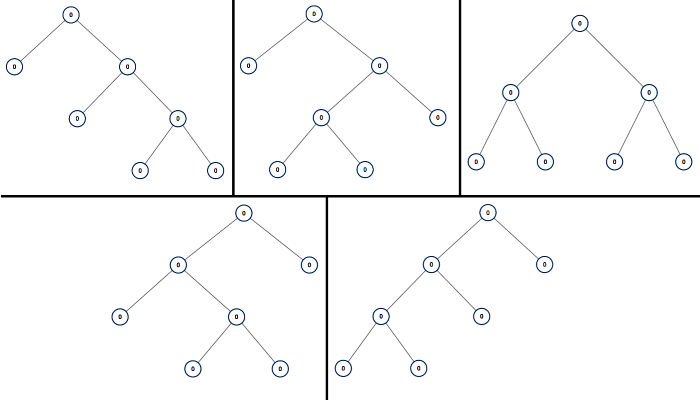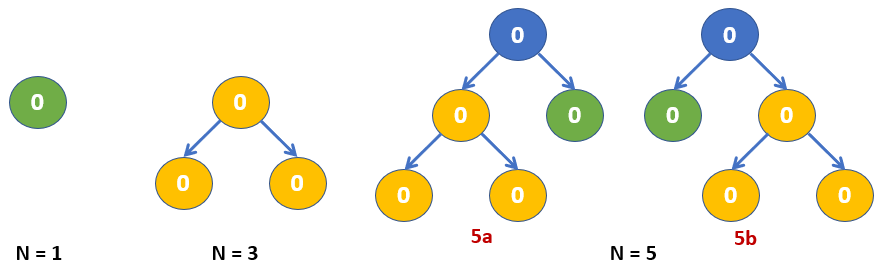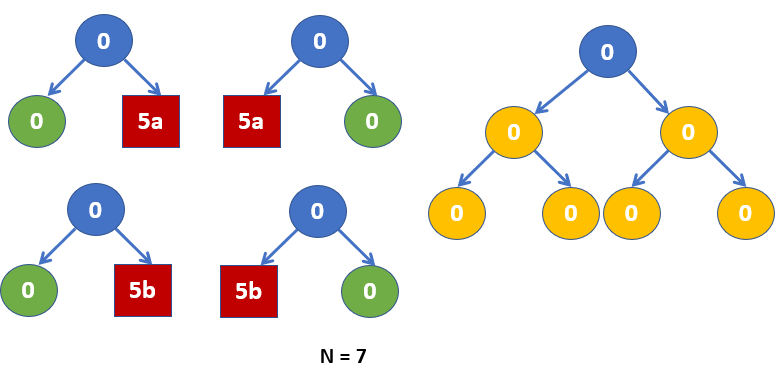# Leetcode — 894. All Possible Full Binary Trees

The link to the problem: https://leetcode.com/problems/all-possible-full-binary-trees/

A full binary tree is a binary tree where each node has exactly 0 or 2 children.

Return a list of all possible full binary trees with `N` nodes. Each element of the answer is the root node of one possible tree.

Each `node` of each tree in the answer must have `node.val = 0`.

You may return the final list of trees in any order.

`Example:Input: 7Output: [[0,0,0,null,null,0,0,null,null,0,0],[0,0,0,null,null,0,0,0,0],[0,0,0,0,0,0,0],[0,0,0,0,0,null,null,null,null,0,0],[0,0,0,0,0,null,null,0,0]]->We will have 5 different kinds of Full Binary Tree as shown in the graph below - `N = 7 (Photo credit to Leetcode.)

# Thoughts:

Let’s draw down the first three cases of N to see if there’s any pattern to generate a full binary tree:Example Full Binary Tree (part 1)

(Since when n=2, 4, or any even numbers, it is impossible for us to create a full binary tree.)

From the above graph, we can see that there’s only one and only possible tree when N = 1 or 3. When N = 5, we can see that the root’s left subtree and right subtree is actually what we will get when N = 1 and N = 3.

Hence, we can also generate all 5 full binary trees with 7 nodes using the trees that we have generated in the above graph. The final result will be shown in the graph below:

⚠️To make the graph clearer, I use 5a and 5b to represent the two different full binary trees of five nodes.Example Full Binary Tree (part 2)

Hence, we can say that if we want to generate a full binary tree with N nodes, we should first know how to generate a full binary tree with N-2, N-4, …, nodes.

Now the problem doesn’t look that hard anymore. As long as we know how we can generate all the possible full binary trees with N-2 nodes, we will know how to generate all possible full binary trees with N nodes.

# Code Logic:

It seems like using recursion to solve this problem will be our best choice. Since we are being asked to design a method that always returns a list of possible full binary trees with the given number of nodes, it means that we can recursively call the same method to help us generate our left subtree and right subtree.

We will need to use a for loop to decide the size of our left subtree and right subtree. Note that the total nodes of the left subtree and right subtree will be n-1 because we will have a root node to connect them.

After we calling allPossibleFBT(i), it means we have found all the roots of the full binary trees with i nodes. Hence, these can all be the root of our left subtree. And the same thing when we call allPossibleFBT(n-i), we also find all the possible roots of our right subtree.
▶️Hence, we will use for loops to generate new root nodes as much as possible until we have tried all the combinations of the left node and the right node.

Below is the 5ms code that beats 23% —

`public List<TreeNode> allPossibleFBT(int n) {        List<TreeNode> rst = new ArrayList<>();        if(n==1)            rst.add(new TreeNode(0));        n-=1;//root        for(int i=1;i<=n;i+=2)        {            List<TreeNode> left = allPossibleFBT(i);            List<TreeNode> right = allPossibleFBT(n-i);            for(TreeNode l: left)            {                for(TreeNode r: right)                {                    TreeNode root = new TreeNode(0);                    root.left = l;                    root.right = r;                    rst.add(root);                }            }        }                return rst;    }`

In the above code logic, it is possible that we spend most of our time finding all the possible trees of the same number of nodes repeatedly. If we use a map to record all the possible trees with a certain number of nodes, we can prevent running the same process again while being asked to return all possible trees with a certain number of nodes.

Below is the 1ms code that beats 99% —

`public List<TreeNode> allPossibleFBT(int n) {        return helper(n, new HashMap<>());    }    public List<TreeNode> helper(int n, Map<Integer, List<TreeNode> > map){        List<TreeNode> rst = map.get(n);        if(rst!=null)            return rst;        rst = new ArrayList<>();        if(n==1)            rst.add(new TreeNode(0));        n-=1;//root         for(int i=1;i<=n;i+=2)        {            List<TreeNode> left = helper(i, map);            List<TreeNode> right = helper(n-i, map);            for(TreeNode l: left)            {                for(TreeNode r: right)                {                    TreeNode root = new TreeNode(0);                    root.left = l;                    root.right = r;                    rst.add(root);                }            }        }        map.put(n+1, rst);        return rst;    }`

--

--